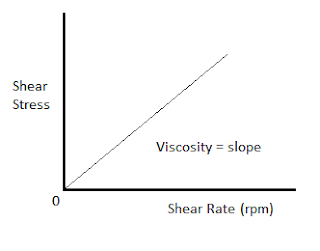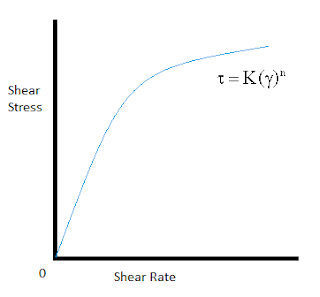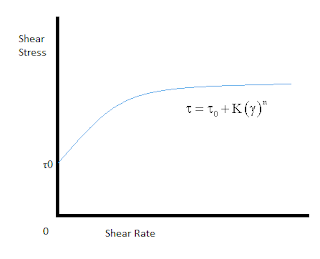# Drilling Fluids Rheological Models

The fluids are categorized into two categories based on their behavior: Newtonian and Non-Newtonian fluids

## Newtonian Fluid

The base fluids used in the compositions of the drilling fluids such as fresh water, sea water, diesel and mineral oils are considered as Newtonian fluids. These fluids are featured by the direct proportion between the shear stress and the shear rate at a constant temperature and pressure. The dynamic viscosity of these fluids is determined by calculation of the slope of the shear stress/shear rate line. This type of fluids are featured also by the zero shear stress for the zero shear rate due to the proportionality between these two parameters which can be noticed on the graph of any Newtonian fluid that starts from origin. Under static conditions, the ability of the Newtonian fluids to suspend cuttings and weighting materials is very weak.

In the case of these types of fluids, the viscosity can be deduced by:

Viscosity (cp) = (Shear Stress / Shear Rate)

Where
Shear stress in  lb/ 100ft²
Shear rate in   1/sFig 1- Newtonian Fluid Profile

## Non-Newtonian Fluids

The Non-Newtonian is characterized by the non-proportional relation between the shear rate and shear stress and they cannot be described by unique viscosity value. Most of the drilling fluids are categorized in this type of fluids due to their complex behavior. The drilling fluids are considered as shear-thinning fluids, that means the effective viscosity decreases with the increase of the shear rate. This characteristic has many benefits:

- Inside the drill string, at high shear rates the viscosity tends to decrease leading to reduced circulating pressures
- In the annulus, the viscosity is at high level due to the low level of shear rates which can help in hole cleaning
- When the circulation is ceased or at very low circulation rate, the drilling fluids develops gel which help to suspend the cuttings and the weighting materials.

There are many models which can describe the relation between the shear rate and shear stress for this type of fluids to understand their behavior. Most used models are Bingham model, Power low model and the Herschel-Bulkley model.

## Bingham Model

This type of fluids obey to a linear relationship between the shear stress and shear rate and requires an initial yield stress before starting to flow, that means when plotting the stress-rate plot, it does not pass through the origin. The Bingham model is applied for laminar flows that means for low shear rates.

The Bingham model can be modeled mathematically by:

τ is the shear stress (lb /100 ft²)
γ is the shear rate  ( 1/s)
YP is the yield stress

PV = 2 x θ(300) – θ(600)

θ : it is the readings at the VG viscometerFig 2- Bingham Model Profile

## Power Law Model

This type of fluids obey to a power law proportionality between the shear rate and shear stress. The equation that can define this behavior is:

K consistency index (fluid pumpability)
n power low index  (degree of Newtonian)

The parameters n and K define the fluid behavior and its level of Non-Newtonian.
The constant 'n' is called the power low index. This index can define the level of the Non-Newtonian behavior. It can take three values:

If n = 1 the fluid is considered as Newtonian
If n<1 the fluid is considered as Non Newtonian
If n>1 the fluid is considered as Dilatant

The constant 'K' is a consistency index and gives the level of the fluid thickness, the higher the K value, the thicker the fluid is.
This model can predict the behavior of drilling fluids better than the Bingham model, but it can show some deviation for low shear rates because this model does not involve the initial yield stress.Fig 3- Power Law Model Profile

## Herschel-Bulkley model (Yield power law model)

This model can predict more accurately the drilling fluids behavior than the other models because it can be considered as the combination of the Bingham model and the Power Law model. This model takes in consideration the weakness of the power law model. It can be modeled as follows:

This model reduces to the Bingham model if n = 1 and to the power law when τ0=0Fig 4- Herschel-Bulkley Model Profile

References
Hussain Rabia. Well Engineering and Construction. 2001
M.E. Hossain & A.A. Al-Majed. Fundamentals of Sustainable Drilling Engineering. Wiley, 2015.